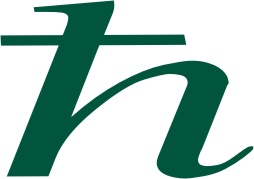Quantum Optics

Course information

Instructor (Prof. Alexander Lvovsky)

Lecture notes

Textbook

Study plan and homework

Week 1

Material covered: Sections 1.6, 2.1 and 2.2 from the book.

Homework for the next week: Exercise 1.21 on p. 23 as well as Problems 2.4 and 2.8 on pages 90-91. Also, you may wish to review the remainder of Chapter 1 and Appendix C.

Week 2

Material covered: Sections 2.3 and 2.6.

Homework for the next week: Please study Sec. 2.3.4 independently and solve end-of-chapter problems 2.11, 2.15, 2.16 as well as Ex. 2.71 on page 89. Also, please review Sec. 5.1 as we will be starting density matrices next week.

Week 3

Material covered: Sections 2.4-2.5, 5.1-5.3.

Homework for the next week: End-of-chapter problems 5.2, 5.4, 5.6. Set up an account on https://quantum-computing.ibm.com/ and solve Problem 10 from here. Next time we will discuss the quantization of the electromagnetic field, so please review Sec. 3.8.1 (harmonic oscillator basics).

Week 4

Material covered: Sec. 3.8 from the book and Sec. 4.1 from the notes

Homework for the next week:

·         Ex. 3.67 (p.137), 3.69 (p.139), 3.75 (p.142) and Problems 3.15, 3.16, 3.17 (p. 165) from the book.

Week 5

Material covered: remainder of Chapter 3 from the book, as well as Secs. 4.4 and 4.6 from the notes

Homework for the next week:

• Problem 6.1 from here.
• Problems 3.18, 3.20 and 3.22 from the book;
• Review Exercises 3.115-3.117 from the book;
• One of the modes of the two-mode squeezed vacuum state with the squeezing parameter r is lost and the state in the remaining mode is analyzed.
• Find the Wigner function of that state.
• Find its density matrix in the position basis.
• Find its photon statistics using the photon statistics of the two-mode squeezed vacuum (can be found in the book).
• Check that the state is thermal [Eq. (4.52) in the notes] and find its temperature parameter b in terms of r.

Week 6

Material covered: Sec. 4.8 from the notes, as well as 4.5 and 5.4 from the book

Homework for the next week:

• Problem 6.3 from here.
• Problem 4.49 from the notes;
• Problems 4.13 and 4.15 from the book;
• Study Sections 4.7.1 and 4.7.2 from the book.

Week 7

Material covered: Sec. 1.4, 1.5, 6.2.1, 6.2.3 from the notes.

Homework for the next week: Problems 1.17, 1.18, 6.18 from the notes.

Week 8

TEST

Tests

2021

2020

2019

Test

Solutions

2018